# VisualizingDendrogramsin R

### Dendro…what?

A dendrogram is the fancy word that we use to name a tree diagram to display the groups formed by hierarchical clustering. If you check wikipedia, you'll see that the term dendrogram comes from the Greek words: dendron=tree and gramma=drawing. There are a lot of resources in R to visualize dendrograms, and in this Rpub we'll cover a broad spectrum of plots so you can have different options from where to choose from the next you want to plot a dendrogram.

### The most basic dendrogram

Let's start with the most basic type of dendrogram. For that purpose we'll use the mtcars dataset and we'll calculate a hierarchical clustering with the function hclust (with the default options).

``````# prepare hierarchical cluster
hc = hclust(dist(mtcars))
# very simple dendrogram
plot(hc)
``````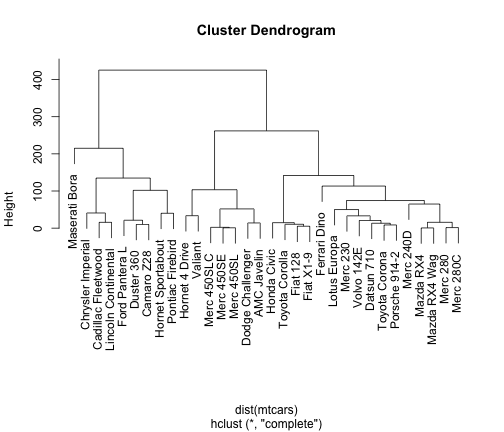We can put the labels of the leafs at the same level like this

``````# labels at the same level
plot(hc, hang = -1)
``````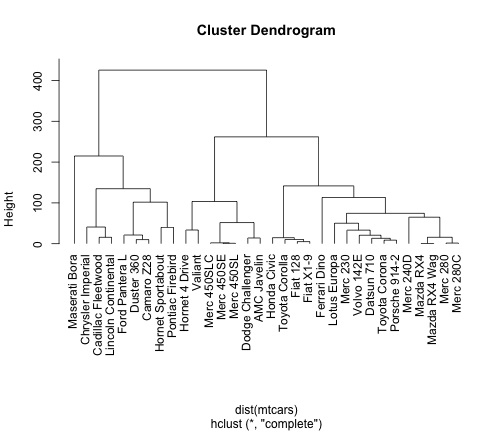### A less basic dendrogram

In order to add more format to the dendrograms, we need to tweek the right parameters. For instance, we could get the following graphic (just for illustration purposes!)

``````# tweeking some parameters
op = par(bg = "#DDE3CA")
plot(hc, col = "#487AA1", col.main = "#45ADA8", col.lab = "#7C8071",
col.axis = "#F38630", lwd = 3, lty = 3, sub = "", hang = -1, axes = FALSE)
axis(side = 2, at = seq(0, 400, 100), col = "#F38630", labels = FALSE,
lwd = 2)
mtext(seq(0, 400, 100), side = 2, at = seq(0, 400, 100), line = 1,
col = "#A38630", las = 2)
``````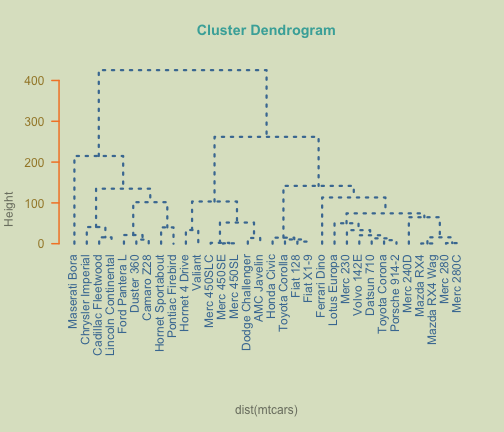``````par(op)
``````

### Alternative dendrograms

An alternative way to produce dendrograms is to specifically convert hclust objects into dendrograms objects.

``````# using dendrogram objects
hcd = as.dendrogram(hc)
# alternative way to get a dendrogram
plot(hcd)
``````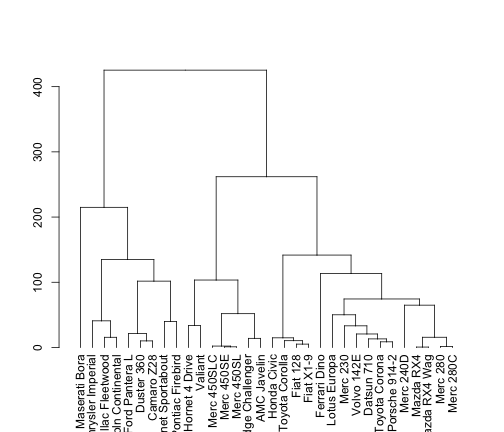Having an object of class dendrogram, we can also plot the branches in a triangular form

``````# using dendrogram objects
plot(hcd, type = "triangle")
``````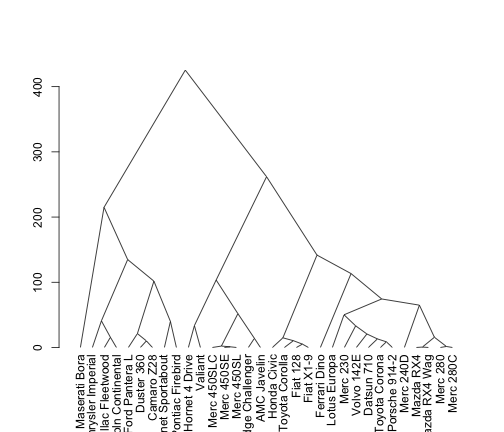### Zooming-in on dendrograms

Another very useful option is the ability to inspect selected parts of a given tree. For instance, if we wanted to examine the top partitions of the dendrogram, we could cut it at a height of 75

``````# plot dendrogram with some cuts
op = par(mfrow = c(2, 1))
plot(cut(hcd, h = 75)\$upper, main = "Upper tree of cut at h=75")
plot(cut(hcd, h = 75)\$lower[], main = "Second branch of lower tree with cut at h=75")
``````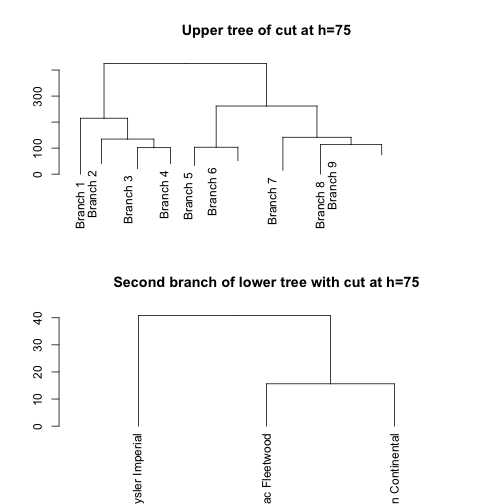``````par(op)
``````

### Customized dendrograms

In order to get more customized graphics we need a little bit of more code. A very useful resource is the function dendrapply that can be used to apply a function to all nodes of a dendrgoram. This comes very handy if we want to add some color to the labels.

``````# vector of colors labelColors = c('red', 'blue', 'darkgreen', 'darkgrey',
# 'purple')
labelColors = c("#CDB380", "#036564", "#EB6841", "#EDC951")
# cut dendrogram in 4 clusters
clusMember = cutree(hc, 4)
# function to get color labels
colLab <- function(n) {
if (is.leaf(n)) {
a <- attributes(n)
labCol <- labelColors[clusMember[which(names(clusMember) == a\$label)]]
attr(n, "nodePar") <- c(a\$nodePar, lab.col = labCol)
}
n
}
# using dendrapply
clusDendro = dendrapply(hcd, colLab)
# make plot
plot(clusDendro, main = "Cool Dendrogram", type = "triangle")
``````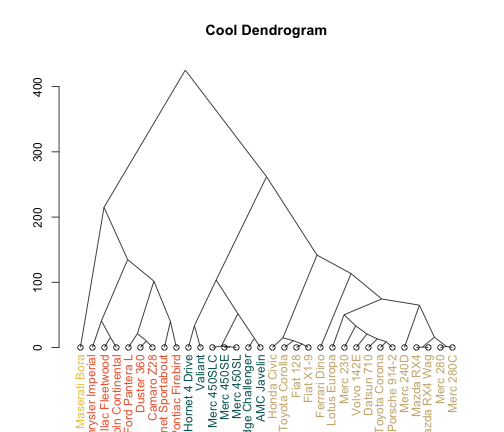### Phylogenetic trees

A very nice tool for displaying more appealing trees is provided by the R package ape. In this case, what we need is to convert the hclust objects into phylo pbjects with the funtions as.phylo

``````# load package ape; remember to install it: install.packages('ape')
library(ape)
# plot basic tree
plot(as.phylo(hc), cex = 0.9, label.offset = 1)
``````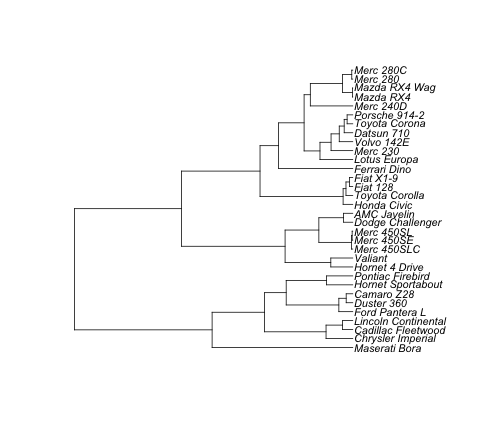The plot.phylo function has four more different types for plotting a dendrogram. Here they are:

``````# cladogram
plot(as.phylo(hc), type = "cladogram", cex = 0.9, label.offset = 1)
``````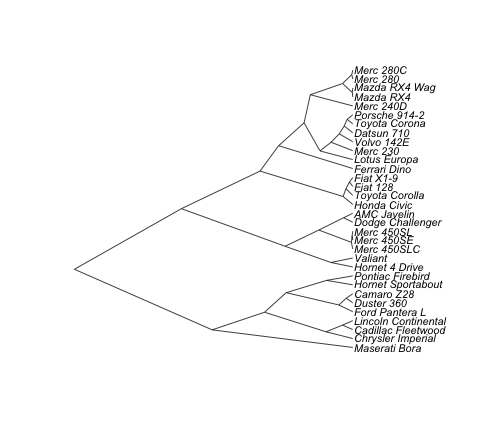``````# unrooted
plot(as.phylo(hc), type = "unrooted")
``````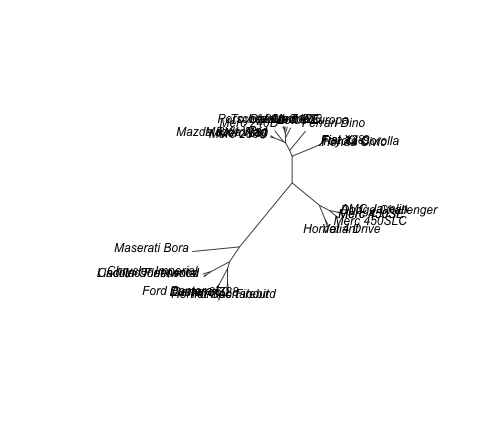``````# fan
plot(as.phylo(hc), type = "fan")
``````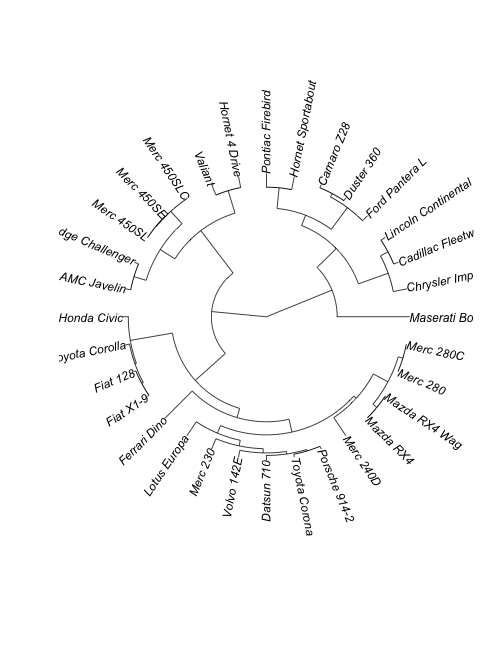``````# radial
``````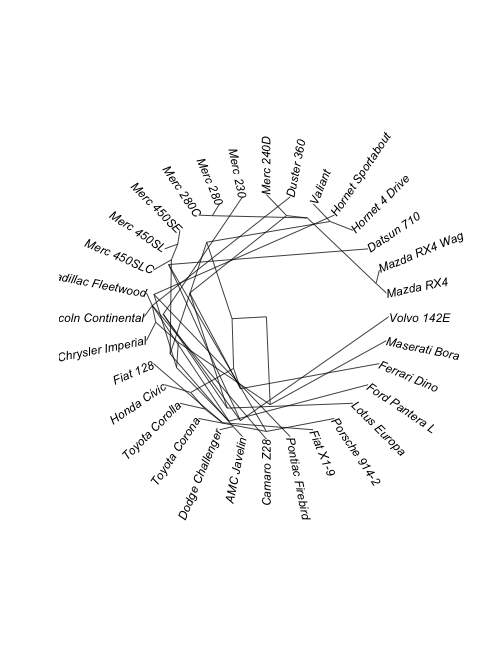### Customizing phylogenetic trees

What I really like about the ape package is that we have more control on the appearance of the dendrograms, being able to customize them in different ways. For example:

``````# add colors randomly
plot(as.phylo(hc), type = "fan", tip.color = hsv(runif(15, 0.65,
0.95), 1, 1, 0.7), edge.color = hsv(runif(10, 0.65, 0.75), 1, 1, 0.7), edge.width = runif(20,
0.5, 3), use.edge.length = TRUE, col = "gray80")
``````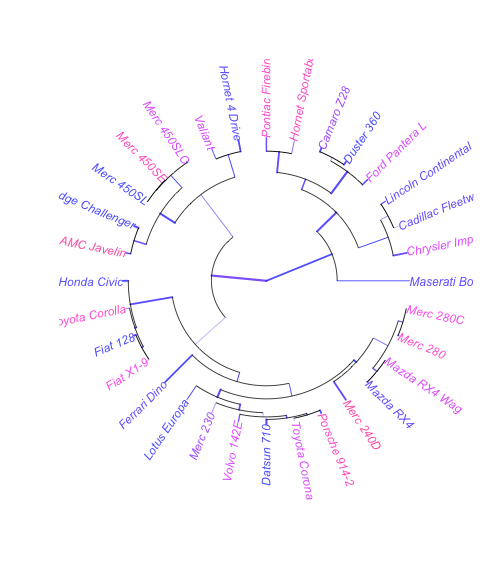Again, we can tweek some parameters according to our needs

``````# vector of colors
mypal = c("#556270", "#4ECDC4", "#1B676B", "#FF6B6B", "#C44D58")
# cutting dendrogram in 5 clusters
clus5 = cutree(hc, 5)
# plot
op = par(bg = "#E8DDCB")
# Size reflects miles per gallon
plot(as.phylo(hc), type = "fan", tip.color = mypal[clus5], label.offset = 1,
cex = log(mtcars\$mpg, 10), col = "red")
``````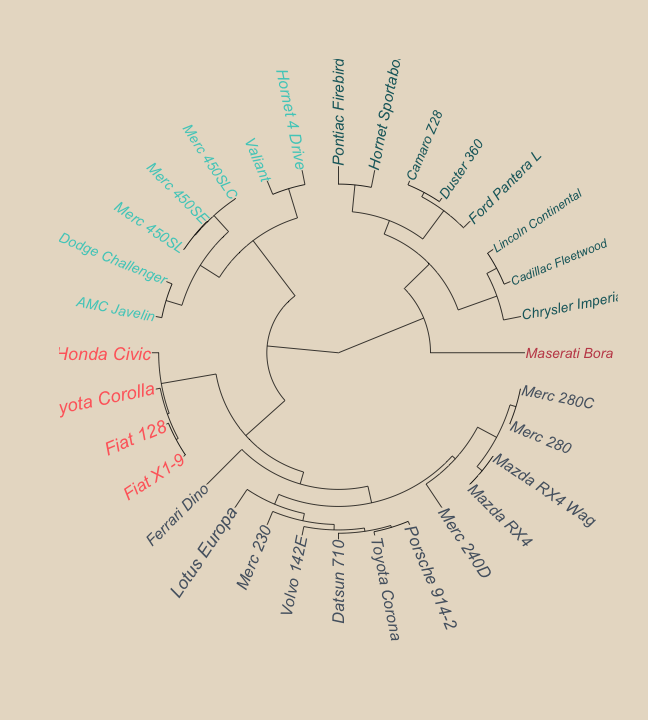``````par(op)
``````

### Color in leaves

The R package sparcl provides the ColorDendrogram function that allows to add some color. For example, we can add color to the leaves

``````# install.packages('sparcl')
library(sparcl)
# colors the leaves of a dendrogram
y = cutree(hc, 3)
ColorDendrogram(hc, y = y, labels = names(y), main = "My Simulated Data",
branchlength = 80)
``````